Courses

# Three Winding Transformer Electrical Engineering (EE) Notes | EduRev

## Electrical Engineering (EE) : Three Winding Transformer Electrical Engineering (EE) Notes | EduRev

The document Three Winding Transformer Electrical Engineering (EE) Notes | EduRev is a part of the Electrical Engineering (EE) Course Electrical Machines.
All you need of Electrical Engineering (EE) at this link: Electrical Engineering (EE)

Three Winding Transformer
In the last class we have analyzed single phase autotransformer, and we have shown that when the requirement of electrical isolation is not very important and the voltage conversion ratio is close to unity, then use of autotransformer offers significant advantage in terms of copper saving, loss saving, etcetera. It is possible to have auto transformer connection for three phase transformers as well.

(Refer Slide Time: 01:09)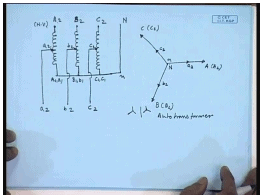For example, let us see a three phase autotransformer connection with high voltage side star connected. This is the neutral where these are the line terminals A 2, B 2, C 2. This is the neutral connection capital N. This is the H.V. side. We can very well have tapings on each individual winding and have terminals a 2, b 2, c 2 and the same terminal as n. So, this is capital A 1 and small a 1; this is capital B 1 and small b 1; this is capital C 1 and small c 1. So, if we draw the line to neutral phasor diagram this is the a phase, this is b phase, this is c phase.

So, this is the point N, this is A that is A 2, this is B that is B 2, and this is C that is C 2. Since, we are putting tapings here the voltages these are the points a 2, b 2, c 2; the voltages a 2 a 1 will be in phase with a 2 a 1. So, this is also the point small n, and this is the point a 2. This is point b 2, and this is point c 2. Hence, we see it is possible to have a three phase star star connected autotransformer.

(Refer Slide Time: 04:31)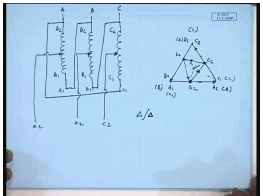Now if we connect the windings in delta that is we again have three H.V windings connected in delta; that is this is A 2 A 1, B 2 B 1, C 2 C 1. A 1 is connected to B 2 in the terminals A, B and C. A 1 is connected to B 2, B 1 is connected to C 2, and C 1 is connected to A 2. The line voltage phasors will be A 2 A 1, B 2 B 1, C 2 C 1. This terminal we are calling A; this terminal we are calling B; this terminal we are calling C. It is junction of A 2 and C 1 is A, B 2 and A 1 is B, and C 2 and B 1 is C.

Now suppose we do the same thing; that is we put certain tapings here. Normally when I have a three phase autotransformer, they are taken from the same percentage tapping of the each winding that is the voltage, and this part, this part, this part are all same in magnitude. Now let us call this a 2, b 2, c 2. So, voltage A 1 small a 2 will be here B 1 small b 2 will be of equal magnitude and C 1 small c 2 will be of equal magnitude. So, the voltages this is the point a 2, this is the point b 2, and this is the point c 2. This is the phasor diagram. We can call these points also small a 1, small b 1, small c 1; that is this point is also a 1, this point is also b 1, and this point is also c 1.

So, the line voltage a 2 b 2, b 2 c 2 and c 2 a 2, a 2 b 2, c 2 a 2 and b 2 c 2 forms a phasor like this. Now when we compare the line to neutral voltage phasors we see that in a star star connected three phase autotransformer the line to neutral phasor, the angle between the line to neutral phasor is 0 irrespective of the position of this taping. So, this is always a star star 0 degree connection; however, when a similar arrangement met with a delta delta connected autotransformer, we see that the line to neutral phasor of the H.V and L.V side, the angle between the line to neutral voltage phasor of the H.V and L.V side actually depends on the position of the taping.

Therefore, if we change this taping this inner triangle will rotate. Hence, the line to neutral voltage phasor of the L.V side and the line to neutral voltage phasor of the H.V sides, the angle between them will depend on the voltage ratio. So, this is one characteristic of delta delta connected thee phase autotransformer; otherwise, the rest of the discussion regarding autotransformer is similar to that of a single phase transformer, and it can be in fact analyzed on per phase basis in a similar way to a single phase transformer single phase autotransformer.

It should be noted, however, that for three phase autotransformer the H.V and L.V side connection are always same; that is it can be only either star star connected or delta delta connected. However, star delta or delta zigzag, delta star, these connections are not possible with three phase autotransformers; however, three phase two winding transformers many of these connections are possible and one such connections we have seen to be the star star connection.

(Refer Slide Time: 11:37)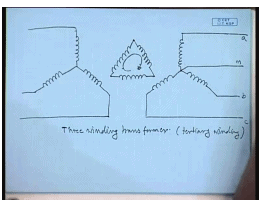That is the high voltage side star connected, low voltage side is also star connected. In many a cases where the low voltage side is supposed to supply unbalanced loads. And in fact single phase load, it is preferable to provide a neutral connection; however, we have observed one problem with this star star neutral connected three phase transformer is that the cohesive currents; that is the zero sequence current of the low voltage side load current and the third harmonic or trip line harmonic currents cannot flow reflection of that current, cannot flow on the high voltage side which has an isolated neutral.

Hence, this produces third harmonic flux distortion. In order to avoid that some path must be provided for the third harmonic current on reflected third harmonic current, so that the third harmonic flux can be canceled. This can be done by a delta connected winding, a third delta connected winding. When we provide a delta connected winding like this, then the cohesive current, the zero sequence and the triplen harmonic currents can circulate within the delta winding thereby canceling the cohesive MMF completely. In many cases such a winding is provided, and those transformers are called the three winding transformer.

So, the third winding is called the tertiary winding, some time also called the stabilizing winding particularly when the purpose of this is to provide a path for the cohesive current. This is called a stabilizing winding; however, it is not essential that this third winding we used only for stabilization purpose, it can be used for other purposes. For example, if this is used in q substation, this third winding can be used to supply auxiliary power which is at a very different voltage than the conversion ratio of this transformer, or this can be used to connect a capacitor bank for supplying leading wire to the bus. Now in this lecture we will see how to analyze this three winding transformer.

(Refer Slide Time: 15:56)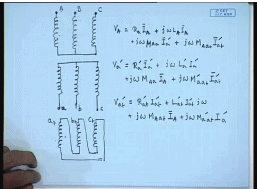For that let us assume that we have a three phase transformer with star star connection of two windings. This is the primary or high voltage winding; this is the low voltage secondary winding, and let us say this is the tertiary winding which is delta connected. Now when we supply sine wave voltage to this winding and load them with a balance load, we have seen that a three phase transformer can be analyzed on per phase basis. Hence by the similar argument, the three winding transformer can also be analyzed per phase basis. And on steady state if we consider a single phase of all the three windings, we can write the applied voltages and the currents as follows by referring everything referring all the voltages to the high voltage side.

Let us say the applied voltage here is V A, and V A can be written as R a into I a. And these are let us say, capital A small a, and a tertiary, b tertiary, c tertiary. V A equal to R a I a plus j omega L A I a plus j omega, mutual inductance between primary and secondary that is M A a into the secondary current reflected to the high voltage side that is I a dash plus j omega, mutual inductance between the high voltage and the tertiary winding that is A a t I a t dash.

Similarly, for the secondary winding referred to the high voltage side can be written as V a dash equal to R a dash I a dash plus j omega L a dash I a dash plus j omega. Now this being symmetrical, this will still be M a a I A plus j omega M dashed a a t I a t dash. And for the tertiary winding we can write V a t dash equal to R a t dash I a t dash plus L a t dash I a t dash j omega plus j omega M A a t I A plus j omega M A a t dash I a dash. Similarly, for other phasors we can write these equations. Hence, we can also write by defining l n to be the magnetizing inductance due to the flux that links all the phasors.

(Refer Slide Time: 23:30)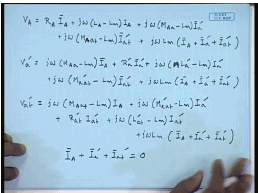We can write V A equal to R A I A plus j omega L A minus L m I A plus j omega M A a minus L m into I a dash plus j omega M A a t minus L m I a t dash. Now the dash refers to the currents referred to the H.V side plus j omega L m into I A plus I a dash plus I a t dash. Similarly, V a dash can be written as j omega M A a minus L m into I A plus R a dash I a dash plus j omega L a dashed minus L m into I a dash plus j omega M a a t dash minus L m I a t dash plus j omega L m I A plus I a dash plus I a t dash.

And v a t dash equal to j omega M A a t minus L m I a plus j omega M A a t dash minus L m into I a dash plus R a t dash I a t dash plus j omega L a t dash minus L m I a t dash plus j omega L m into I a plus I a dash plus I a t dash. Now if we assume that the magnetization current of this transformer is negligibly small, then we have I a plus I a dash plus I a t dash equal to 0.

(Refer Slide Time: 29:17)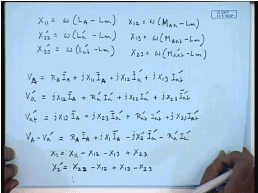Let us also define X 11 to be equal to omega into L A minus L m. X 22 dashed equal to omega into L a dash minus L m. X 33 dashed equal to omega into L a t dash minus L m. Similarly, let us define X 12 to be equal to omega into M A a minus L m. X 13 equal to omega into M A a t minus L m and X 23 equal to omega into M A a t dashed minus L m. Then we can write V a equal to R A I A plus j X 11 I A plus j X 12 I a dash plus j X 13 I a t dash. V a equal to j X 12 I A plus R a dash I a dash plus j X 22 I a dash plus j X 23 I a t dash.

And V a t dashed equal to, V a t dashed can be written as j X 13 I A plus j X 23 I a dash plus R a t dash I a t dash plus j X 33 I a t dashed; from which we can further simplify it to V A minus V a dash equal to R A I A plus j X 1 I A minus j X 2 dash I a dash minus R a dashed I a dash, where we have defined X 1 to be equal to X 11 minus X 12 minus X 13 plus X 23. And X 2 dash has been defined as X 23 minus X 12 plus X 13, sorry X 22, minus X 23.

(Refer Slide Time: 36:15)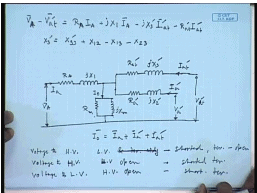Similarly, we can find out V A minus V a t dash. This is given by R A I A plus j X 1 I A minus j X 3 dash I a t dash minus R a t dash I a t dash, where X 3 dash has been defined as X 33 dash plus X 12 minus X 13 minus X 23. These equations then suggests the flowing equivalent circuit for the three winding transformer. This voltage is V A, this voltage is V a t dash, and this voltage is V a dash. Hence, this impendence is R a t dashed. This is minus; this is j X 3 dash. This is R a dash, this is j X 2 dash; current flowing into this circuit is I a, current flowing into this circuit it is I a t dash, and the current flowing into this circuit is I a dash.

This circuit is completed by putting a magnetization branch just like a two winding transformer like this also referred to the high voltage side. This is R m, this is j X m, and this is I 0, where I 0 equal to I a plus I a dashed plus I a t dash. So, this is the equivalent circuit of a three winding transformer. The shunt branch parameter as usual can be determined from the open circuit test that is applying rated voltage to any one of the three windings and keeping the other windings open. Similarly, the series branch parameter there are these parameters can be obtained from short circuit test; however, here three sets of short circuit test are to be conducted.

One set will be voltage to H.V side, L.V and tertiary. This will be shorted. Next you apply voltage to L.V side, H.V, and sorry, in this case you have to do three sets of short circuit test. One, voltage to H.V side, L.V side shorted, tertiary winding open. Then voltage to H.V side L.V side open and shorted is tertiary winding. Then voltage to L.V winding, H.V winding shorted. H.V winding is left open and short circuited tertiary winding. From these three sets of short circuit test data, we can find out the parameters of a three winding transformer. Let us see how through an example.

(Refer Slide Time: 44:07)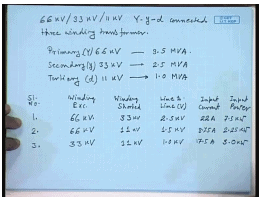Another side we have a 66 kV by 33 kV by 11 kV Y y d connected three winding transformer. Then the winding voltage and KVA ratings are as follows. The H.V winding or the primary winding that is 66 kV and KVA rating is 3.5 MVA. Secondary this is y connected; this is also y connected. This is 33 kV, and its KVA rating is 2.5 MVA. The tertiary this is delta connected; this is 11 kV, and its MVA rating is 1.0 MVA. In order find out the series branch parameter of this three winding transformer, the following test are created are conducted.

So, serial number, then winding excited, and the winding shorted the line to line voltage of excitation voltage, input current standard data for a short circuit test, input power. The serial number one, winding excited is a 66 KVA winding, the shorted winding is the 33 kV, the applied line to line voltage on the 66 kV is 2.5 kV; that circulates a current of 22 ampere secondary winding, and the input power is 7.5 kilowatt. The second test again the excited winding is the 66 kV winding. However, this time the shorted winding is the 11 kV winding needed to apply 1.5 kilovolt to circulate a current of 8.75 ampere, and the input power was 2.25 kilowatt.

In the third test the excited winding now is the 33 kV, and the shorted winding is 11 kV. Applied voltage is 1 kV, the current circulating the 33 kV winding is 17.5 ampere, and the input power is 3.0 kilowatt. Now referring to this test data we can see if we keep a winding open, then that resistance does not come into consuming power from the input power. And if we neglect the magnetization branch during short circuit test, then the total power that is consumed is consumed in the two resistances of the three winding transformer.

And with one of the winding open, these two currents are almost same in magnitude, because this winding is open and the no load current where assuming to be negligible. Hence, for the first test data the input power of 7.5 kilowatt is consumed in the high voltage winding and in the 33 kV winding; that is in this winding and in this winding that is in this resistance and in this resistance. So, the equivalent resistance of the 66 kV and the 33 kV windings referred to the 33 kV side will be.

(Refer Slide Time: 50:32)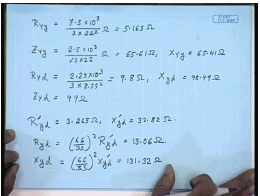Let us call it R Y y; this should be equal to 7.5 into 10 to the power 3 divided by 3 into the circulated current is 22 amperes. This gives me a value of 5.165 ohm. By the similar argument, the impendence z Y y will be equal to 2.5 kV. This gives me 65.61 ohm. Hence, X Y y equal to 65.41 ohm. Similar arguments R y d equal to 2.25 into 10 to the power 3 divided by 3 into 8.75 square that comes to 9.8 ohm. And X y d comes to be 98.49 ohm. Z Y d is 99 ohm.

R dashed y d it is referred R dash means referred to the 66 kV winding; R dashed means referred to the 33 kV winding. This comes to 3.265 ohm, and X y d dash comes to be 32.83 ohm. Now these are to be referred to the 66 kV winding that is R y d equal to 66 by 33 whole square R y d dash. This gives me 13.06 ohm. Similarly, X y d is 66 by 33 whole square X y d dash. This comes to 131.32 ohm.

(Refer Slide Time: 54:21)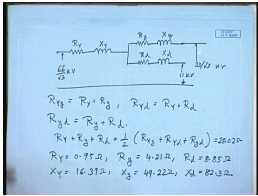Now generally equivalent circuit of a three winding transformer as we have seen earlier is something like this. This is R capital Y; this is X capital Y; this is R small y; this is X small y; this is R small d; this is X small d. This voltage is 66 by root 3 kV. This voltage is 33 by root 3 kV; this is 11 kV. Now R Y y equal to R Y plus R small y. Similarly R Y d equal to R capital y plus R small d, and R small y small d equal to R small y plus R small d; therefore, R capital y plus R small y plus R small d equal to half of R Y y plus R Y d plus R small y small d. This comes to 28.02 ohms.

Hence, from here we can find out R Y equal to 0.95 oh because we known R capital Y y, R small y comes to 4.21 ohm and R small d comes to 8.85 ohm. In a similar manner X Y comes to 16.39 ohm. X small y comes to 49.22 ohm, and X small d to 82.3 ohm. Hence, we see that from a set of three short circuit test data we can find out the equivalent circuit parameters of a three winding transformer which can later be used in the usual manner to solve or analyze different operating situations in the three winding transformer.

Thank you.

Offer running on EduRev: Apply code STAYHOME200 to get INR 200 off on our premium plan EduRev Infinity!

61 docs|20 tests

,

,

,

,

,

,

,

,

,

,

,

,

,

,

,

,

,

,

,

,

,

;